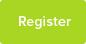# Numerical Solutions Of Equations

Mathematical research into the nature of equations that cannot be solved analytically

Date : 26/6/2016### Author Information

Uploaded by : Siling
Uploaded on : 26/6/2016
Subject : Maths

### Introduction

In order to solve equations, sometimes a numerical approach must be taken since they cannot be solved analytically, such as by factorising or by using the formula, as in the case with quadratics. For functions with higher orders and that do not factorise, they must be solved numerically. For equations that do not factorise, a graphical representation of the equation is needed to find the best approximation of non-integer values for the roots. These can be found by a number of different numerical methods.

There will be three different numerical methods used here to solve different equations. They are the Decimal Search method, the Newton-Raphson method and lastly a Fixed-point Iteration method sometimes known as the Rearranging method.

### The Decimal Search Method

This method involves finding a root when there is a change of sign.

Application of the method to find the first root

The graph shown above is 𝑦& = 𝑓& 𝑥lt;br> Where 𝑓&𝑥& =𝑥&!−&7𝑥&+2

From the graph there appears to be a root at interval [2,3]. We can test this using the change of sign method:

Root to be found using this method

𝑓& 2 = −&4

𝑓&3=8

The change of sign confirms there is a root because the root of this equation is the point at which the graph of y=f(x) crosses the x-axis, which is when f(x) changes sign.
We then split this interval into ten sections, and test each for a change of sign, and continue this until an interval with enough precision is obtained to find the root.

1

Interval [2, 3]

Since the change of sign occurs in the interval [2.4, 2.5], the root is also in this interval.

Interval [2.4, 2.5]

The change of sign occurs in the [2.48, 2.49] interval, so the root is in this interval.

2

Interval [2.48, 2.49]

Interval [2.489, 2.490]

The change of sign occurs in the [2.4892, 2.4893] interval. We now take the midpoint of the interval as the value for the root.

Therefore 𝑥& = 2.48925 with a maximum error of ±&0.00005

Failure of the Decimal Search Method to find a root

3

The graph shown above is 𝑦& = 𝑓&(𝑥&)
where 𝑓& 𝑥& = 5𝑥&! −& 4.2𝑥&! −& 18.318𝑥& + 20.164

So we are trying to find the roots of the equation 5𝑥&! −& 4.2𝑥&! −& 18.318𝑥& + 20.164 = 0 From the graph, there appears to be a root in the interval [1, 2], so we should check for

There is no change of sign in this interval, and so this method fails here, even though the graph shows there is a root in this interval.

The equation can be factorised into the form 0 = 5(𝑥& + 2)(𝑥& −& 1.42)!.

Therefore we can clearly see that the root in the interval [1, 2] is 𝑥& = 1.42. However, since the term is squared, the root is repeated, and so never crosses the x-axis. Therefore there is no change of sign and this method does not work.

4

Newton-Raphson Method

The image above shows the graph of𝑦&=𝑥&! −&5𝑥&! +2𝑥&+4, and so we will be using the Newton-Raphson iterative method to solve the equation 𝑓& 𝑥& = 0 where𝑓& 𝑥& =𝑥&! −&5𝑥&! +2𝑥&+4.

This method makes use of the iterative formula 𝑥&!!! = 𝑥&! −& !(!!) . !!(!!)

Therefore,weneedtofind𝑓&! 𝑥& = ! 𝑥&! −&5𝑥&! +2𝑥&+4

𝑓&! 𝑥& =3𝑥&!−&10𝑥&+2

And so the iterative formula is 𝑥&!!! = 𝑥&!

Root 1

The first root to be found is the root in the interval [4, 5], and so we will take x0 = 5. Putting this into the iterative formula, Excel produces the following results:

Roots to be found using this method

5

X2

X1 X0

This iterative method works by drawing a tangent to the graph of y=f(x) at the point (x0, f(x0)), and then making the next x value, x1, the point at which this tangent crosses the x-axis. This method is then repeated for x1 and x2 until the value for xr is obtained to the required degree of accuracy.

The method clearly converges on a root at x=4.323404. We can test for a root here by checking for a change of sign on either side of this value.

As f(x) changes sign, there is a root inside this interval. Therefore, 𝑥& = 4.323404 with a maximum error of ±& 0.0000005.

Root 2

The next root to be found is in the interval [1, 2], so we will take x0 = 1.

The method clearly
converges on a value,
so we can test this
with a change of sign
test to confirm there is a root there.

Therefore, 𝑥& = 1.357926 with a maximum error of ±&0.0000005

6

Root 3

The final root to be found with this method is the one in the interval [-1, 0], and so we will take x0 to be -1.

The method converges clearly on a root, so we must check for a change of sign above and below the root, to ensure that a root is present.

There is an obvious change of sign, so
𝑥& = −&0.681331 with a maximum error of ±&0.0000005

Failure of the method

The image above shows the graph of𝑦&=𝑓& 𝑥& where𝑓& 𝑥& =𝑥&! −&𝑥&! −&5𝑥&+1, and so we will attempt to find a root of the equation 𝑓& 𝑥& = 0.

For this method, we need to find 𝑓&′&(𝑥&), which we will do by differentiating the function:

Root to be found with this method

7

𝑓&𝑥& =𝑥&!−&𝑥&!−&5𝑥&+1 𝑓&! 𝑥& =3𝑥&!−&2𝑥&−&5

Therefore the iterative formula is 𝑥&!!! = 𝑥&! −& !!!!!!!!!!!!! !!!!!!!!

This resource was uploaded by: Siling